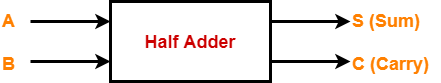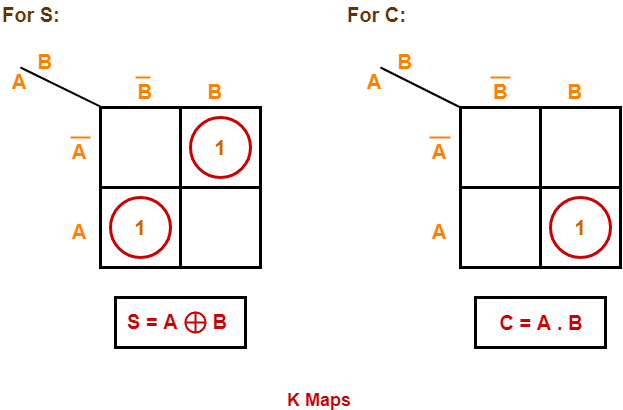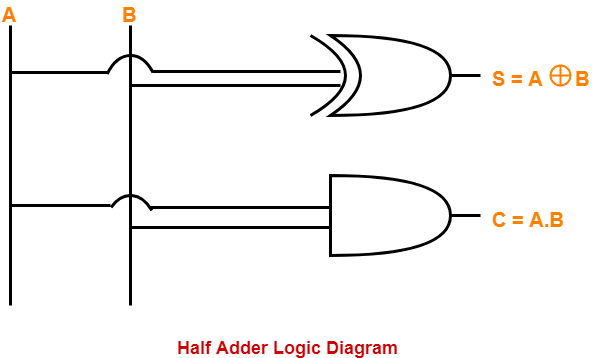# Half Adder | Definition | Circuit Diagram | Truth Table

• Half Adder is a combinational logic circuit.
• It is used for the purpose of adding two single bit numbers.
• It contains 2 inputs and 2 outputs (sum and carry).## Half Adder Designing-

Half adder is designed in the following steps-

### Step-01:

Identify the input and output variables-

• Input variables = A, B (either 0 or 1)
• Output variables = S, C where S = Sum and C = Carry

### Step-02:

Draw the truth table-

 Inputs Outputs A B C (Carry) S (Sum) 0 0 0 0 0 1 0 1 1 0 0 1 1 1 1 0

### Step-03:

Draw K-maps using the above truth table and determine the simplified Boolean expressions-Also Read- Half Subtractor

### Step-04:

Draw the logic diagram.

The implementation of half adder using 1 XOR gate and 1 AND gate is as shown below-## Limitation of Half Adder-

• Half adders have no scope of adding the carry bit resulting from the addition of previous bits.
• This is a major drawback of half adders.
• This is because real time scenarios involve adding the multiple number of bits which can not be accomplished using half adders.

To overcome this drawback, Full Adder comes into play.

To gain better understanding about Half Adder,

Watch this Video Lecture

Next Article- Full Adder

Get more notes and other study material of Digital Design.

Watch video lectures by visiting our YouTube channel LearnVidFun.

SummaryArticle Name
Half Adder | Definition | Circuit Diagram | Truth Table
Description
Half Adder is a combinational logic circuit used for the purpose of adding two single bit numbers. Half Adder Definition, Block Diagram, Truth Table, Circuit Diagram, Logic Diagram, Boolean Expression and Equation are discussed.
Author
Publisher Name
Gate Vidyalay
Publisher Logo